## Diophantine Equation--7th Powers

The 2-1 equation(1)

is a special case of Fermat's Last Theorem with, and so has no solution. No solutions to the 2-2 equation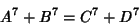(2)

are known.

No solutions to the 3-1 or 3-2 equations are known, nor are solutions to the 3-3 equation(3)

(Ekl 1996).

No 4-1, 4-2, or 4-3 solutions are known. Guy (1994, p. 140) asked if a 4-4 equation exists for 7th Powers. An affirmative answer was provided by (Ekl 1996),(4)(5)

A 4-5 solution is known.

No 5-1, 5-2, or 5-3 solutions are known. Numerical solutions to the 5-4 equation are given by Gloden (1948). The smallest 5-4 solution is(6)

(Lander et al. 1967). Gloden (1949) gives parametric solutions to the 5-5 equation. The first few 5-5 solutions are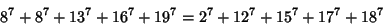(7)(8)(9)(10)(11)

(Lander et al. 1967).

No 6-1, 6-2, or 6-3 solutions are known. A parametric solution to the 6-6 equation was given by Sastry and Rai (1948). The smallest is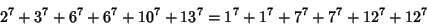(12)

(Lander et al. 1967).

There are no known solutions to the 7-1 equation (Guy 1994, p. 140). A 2-10-10 solution is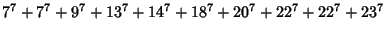(13)

(Lander et al. 1967). The smallest 7-3 solution is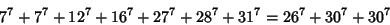(14)

(Lander et al. 1967).

The smallest 8-1 solution is(15)

(Lander et al. 1967). The smallest 8-2 solution is(16)

(Lander et al. 1967).

The smallest 9-1 solution is(17)

(Lander et al. 1967).

References

Ekl, R. L. Equal Sums of Four Seventh Powers.'' Math. Comput. 65, 1755-1756, 1996.

Gloden, A. Zwei Parameterlösungen einer mehrgeradigen Gleichung.'' Arch. Math. 1, 480-482, 1949.

Guy, R. K. Sums of Like Powers. Euler's Conjecture.'' §D1 in Unsolved Problems in Number Theory, 2nd ed. New York: Springer-Verlag, pp. 139-144, 1994.

Lander, L. J.; Parkin, T. R.; and Selfridge, J. L. A Survey of Equal Sums of Like Powers.'' Math. Comput. 21, 446-459, 1967.

Sastry, S. and Rai, T. On Equal Sums of Like Powers.'' Math. Student 16, 18-19, 1948.